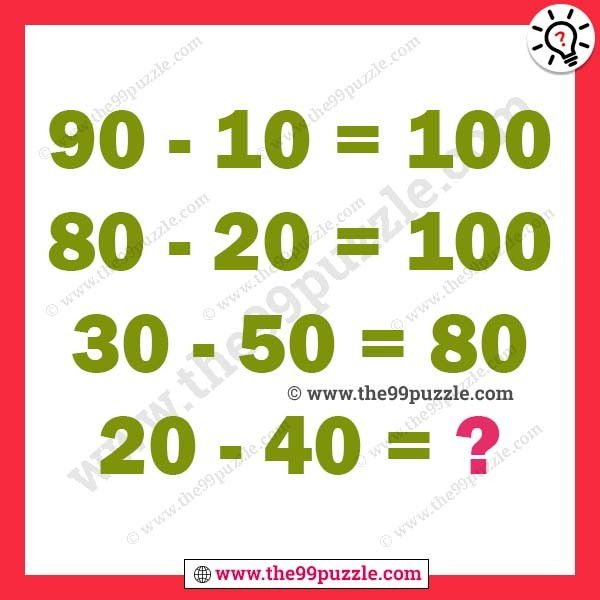# Maths logical reasoning questions with answers – Puzz175

Maths logical reasoning questions with answers. This is a very interesting maths logical equations puzzle that will challenge your brain. In this math logic puzzle, you are shown some logical number equations. Your challenge is to find the logic of the logical equation and find the missing number which will replace the question mark.

90−10=100

80−20=100

30−50=80

20−40=?###### Explanation:

90−10 = (9+1) = 10 0

80−20 = (8+2) = 10 0

30−50 = (3+5) = 8 0

20−40 = (2+4) = 6 0

1.